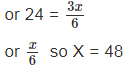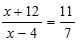CLAT  >  Solved Examples of Ages

# Solved Examples of Ages - Notes | Study Quantitative Techniques for CLAT - CLAT

 1 Crore+ students have signed up on EduRev. Have you?

Solved Examples of AGES

Example 1So man’s age is 48 years

Example 2: Difference of ages of A and B is 16 years. Four years ago the ratio of ages of A and B was 11 : 7. What is the present age of B.

Solution: Let the age of B be x

So A’s age was x + 16 – 4 = X + 12

Four years ago B’s age was X – 4

As per the statement four years ago the age of A:

Age of B was 11:7

So formulating the equationCross multiply 7 X + 84 = 11 X – 44

or 128 = 4x

or X = 32 So B’s present age is 32 yrs.

The document Solved Examples of Ages - Notes | Study Quantitative Techniques for CLAT - CLAT is a part of the CLAT Course Quantitative Techniques for CLAT.
All you need of CLAT at this link: CLAT

## Quantitative Techniques for CLAT

56 videos|35 docs|91 tests

## Quantitative Techniques for CLAT

56 videos|35 docs|91 tests

Track your progress, build streaks, highlight & save important lessons and more!

,

,

,

,

,

,

,

,

,

,

,

,

,

,

,

,

,

,

,

,

,

;